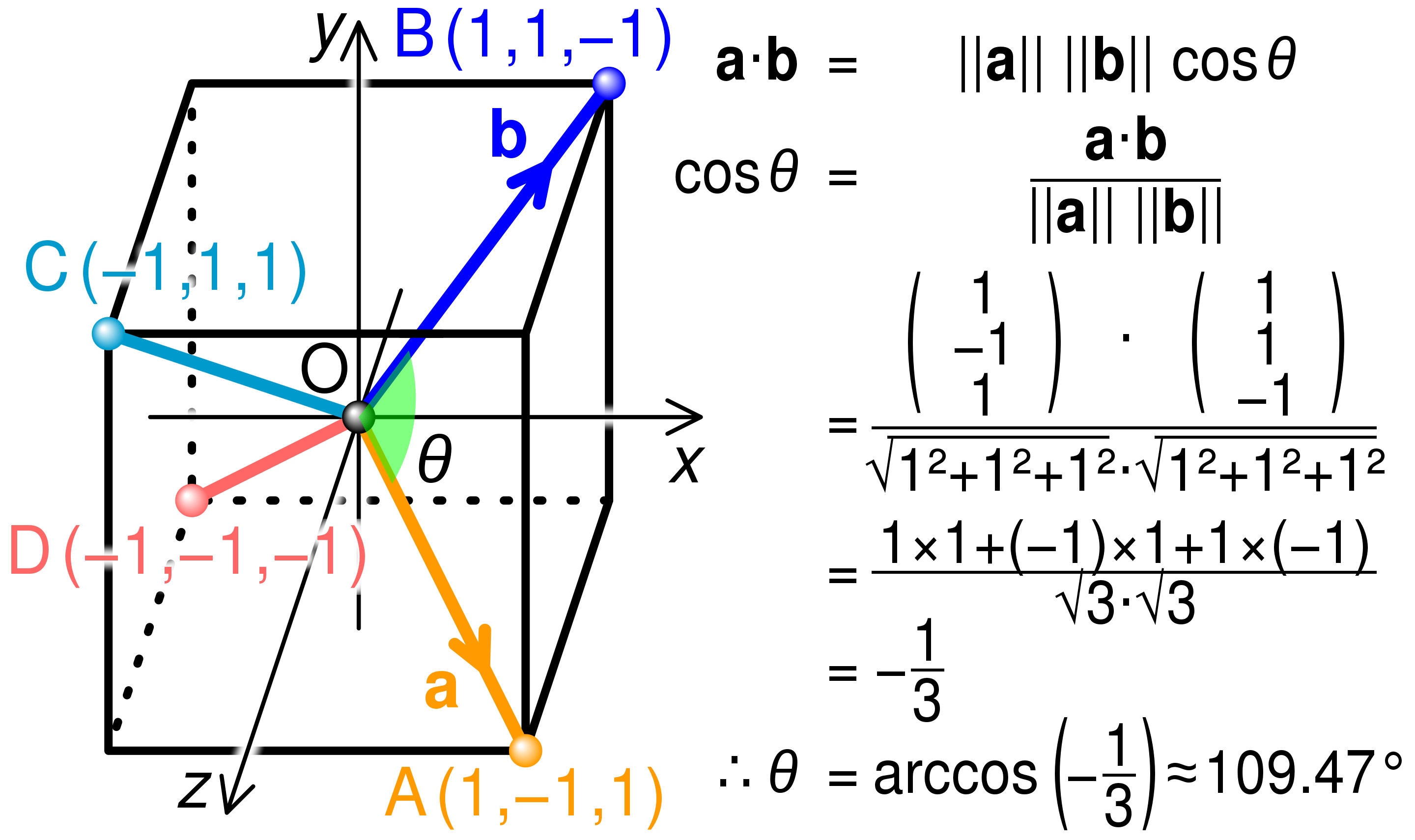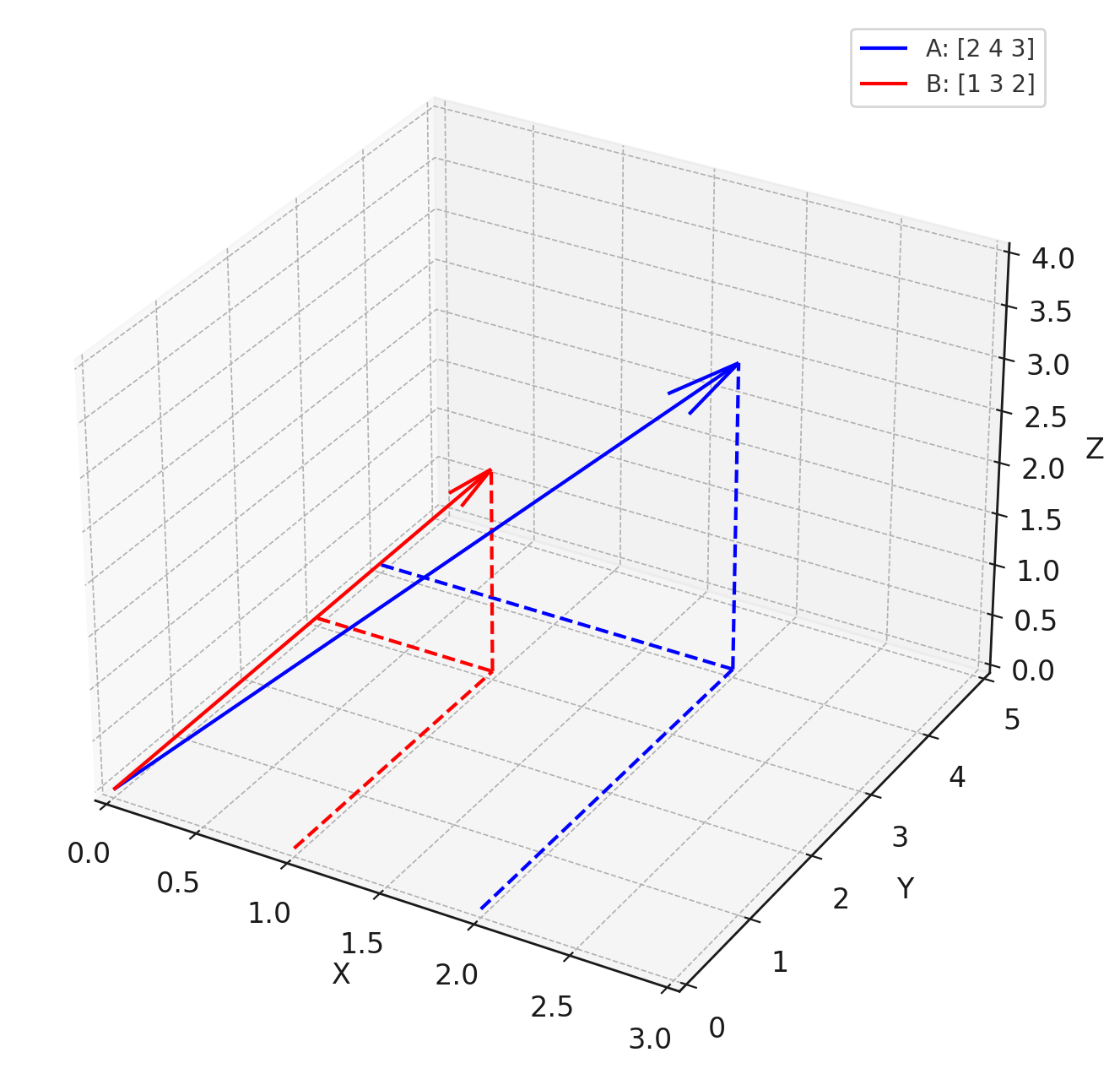• 怎么理解这里的相似？
• 为啥要用点积衡量相似度？
• 怎么理解多维向量？
• 向量是怎么得出来的？
• 具体计算步骤是怎么样的？
• 应用场景有哪些？

## 怎么理解相似？

• 方向：向量的方向是相似度最直观的一面。在二维或三维空间里，你可以直观地想象两个向量是否大致指向同一方向。如果两个向量之间的夹角趋近于0度，那么它们就被认为是非常相似的。
• 长度：向量的长度（或模）也可以是衡量相似度的一个因素，尤其是在需要考虑“强度”或“大小”的场合。然而，在很多应用中，例如文本相似度计算，通常会忽略向量长度的影响，只考虑方向。
• 度量方法：不同的度量方法会给出不同类型的相似性信息。除了点积外，还有余弦相似度、欧氏距离、曼哈顿距离等多种方法。
• 点积: 如前所述，主要衡量方向和长度。
• 余弦相似度(Cosine Similarity): 只考虑方向，不考虑长度。是点积的一个归一化版本。
• 欧氏距离: 衡量两点之间的“距离”，而非方向。

## 为啥用点积衡量？

• 夹角的度量：点积与两向量之间的夹角有关。当两个向量的方向完全相同（即夹角为0度）时，点积最大。反之，当两个向量正交（即夹角为90度）时，点积为零。
• 长度的影响：点积同时考虑了向量的长度和方向。因此，长的、方向相似的向量会有更大的点积。
• 计算简便：点积计算相对简单，只涉及基础的算术运算，这使得它在大规模数据处理中非常实用。
• 可解释性：点积的结果可以轻易地通过夹角和长度进行解释，这在许多应用场景（如自然语言处理、推荐系统等）中是有用的。
• 线性性质：点积满足分配律和结合律，这意味着它可以轻易地应用于更复杂的数学结构和算法中。

## 怎么理解多维?

$$A \cdot B = \sum_{i=1}^{n} a_i \times b_i$$

$$| A | = \sqrt{\sum_{i=1}^{n} a_i^2} , \quad | B | = \sqrt{\sum_{i=1}^{n} b_i^2}$$

$$\cos(\theta) = \frac{A \cdot B}{| A | \times | B |}$$

## 怎么找到合适的向量？

• 词频（TF）：仅使用词频（Term Frequency）来表示文本。
• TF-IDF（Term Frequency-Inverse Document Frequency）：使用词频（TF）和逆文档频率（IDF）的乘积来表示文本。

• Word2Vec：利用神经网络模型来构建高维的词向量。
• GloVe（Global Vectors for Word Representation）：通过全局统计信息来构建词向量。
• FastText：与 Word2Vec 类似，但考虑了词内的子结构（如字符 n-grams）。

• BERT（Bidirectional Encoder Representations from Transformers）：使用 Transformer 模型，并考虑了上下文信息。
• GPT（Generative Pre-trained Transformer）：类似于 BERT，但通常用于生成任务。

## 具体计算步骤### 人工计算

$\mathbf{A} \cdot \mathbf{B} = a_1 \cdot b_1 + a_2 \cdot b_2 + \ldots + a_n \cdot b_n$

$\mathbf{A} \cdot \mathbf{B} = 2 \times 1 + 4 \times 3 + 3 \times 2 = 2 + 12 + 6 = 20$

$\text{Cosine Similarity} = \frac{\mathbf{A} \cdot \mathbf{B}}{| \mathbf{A} | \times | \mathbf{B} |}$

$| \mathbf{A} | = \sqrt{a_1^2 + a_2^2 + \ldots + a_n^2}$
$| \mathbf{B} | = \sqrt{b_1^2 + b_2^2 + \ldots + b_n^2}$

$\text{Cosine Similarity} = \frac{20}{\sqrt{2^2 + 4^2 + 3^2} \times \sqrt{1^2 + 3^2 + 2^2}} \approx \frac{20}{\sqrt{29} \times \sqrt{14}} \approx 0.993$

## 应用场景

• 文本相似性和文档检索：通过计算文档或句子的词向量（通常是TF-IDF或词嵌入向量）的点积或余弦相似度，可以快速找出内容相似的文档或句子。这在信息检索、文档分类和聚类等任务中非常有用。
• 词义相似性：词嵌入技术（如Word2Vec、GloVe等）生成的词向量可以用点积或余弦相似度来衡量词义的相似性或相关性。
• 机器翻译：在序列到序列的模型，比如 Transformer 中，注意力机制经常使用点积来计算不同词或短语之间的关联强度。
• 情感分析：在情感分析中，可以通过计算文本与预定义情感词的点积或余弦相似度，来评估文本的情感倾向。